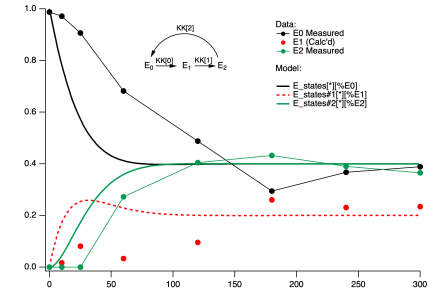# fitting parameters for a system of differential equationsI have defined a system of differential equations controlled by three parameters. it describes the time evolution of 3 species defined by 3 rate constants KK, KK and KK. The model is supposed to describe data that is presented in graph zero as lines and markers (the data describes the species labeled E0 and E2. E1, in this case, is just obtained by assuming E0 + E1 + E2 = 1). my goal here is to vary the KK parameters to get as close as possible to the data. ( I realize the fit will may not be great, and getting a good fit may require a more sophisticated model, just want to see how closely this model can fit)  How can i do this with Igor? ihave attached the relevant pxp file which has all the data and the model in it.Fitting two components is a bit tricky, start by fitting one component. I've looked at your experiment file and I'm a little bit confused about your intentions; it looks like you started from the fit function in the Differential Equation Demo experiment, which is too simple for your case.

You have three rate constants- if they are independent, then you have three fit coefficients just for the rate constants. It looks like the initial conditions are simply E0=1, E1=E2=0, so no need to put those into the fit coefficients. It's not clear that you need fit coefficients other than your rate constants. Here is a possible fit function for fitting the third component:

Function Fit_Chem_kin(pw, yw, xw) : FitFunc
wave pw, yw,  xw

// pw contains the rate constants

Make/FREE/D/N=(numpnts(yw), 3) ODEsolution
ODEsolution = 1
ODEsolution = 0
ODEsolution = 0
IntegrateODE /X=xw chem_kin,  pw, ODEsolution       // we use pw as the parameter wave for IntegrateODE if the rate constants are the only fit coefficients
yw = ODEsolution[p]                          //  if we are fitting the third component
End

A flexible but inefficient way to fit the first and third components would be to write two fit functions, one for each component. Then use the Global Fit package to fit the two components together. That will evaluate the ODE twice as many times as necessary, though.

Another way to fit two components would be to concatenate the two components into a single wave, one after the other. The wave would have 2N components, the first N would be the first component, the second N would be the third component. You also need to make an X wave that has all the X values twice, one set for the first component, one for the third. The fit function might look like this:

Function Fit_Chem_kin_2Components(pw, yw, xw) : FitFunc
wave pw, yw,  xw

// pw contains the rate constants
Variable npnts = numpnts(yw)
Make/FREE/D/N=(npnts/2, 3) ODEsolution
Duplicate/FREE/R=[0,npnts/2-1] xw, ODEx             // just need half the X values for the ODE solution
ODEsolution = 1
ODEsolution = 0
ODEsolution = 0
IntegrateODE /X=ODEx chem_kin,  pw, ODEsolution     // we use pw as the parameter wave for IntegrateODE if the rate constants are the only fit coefficients
yw[0,npnts/2-1] = ODEsolution[p]                         // first component
yw[npnts/2, npnts-1] = ODEsolution[p]                    // third component
End

FuncFit doesn't know that your fit function expects two data sets in the yw wave, so the /D (autodestination) doesn't work. You can use /D=destwave, though, because all the numbers of points and point order will Just Work that way.

Good luck with this project, and feel free to ask more questions. I guarantee you will have more :)ForumSupportGallery